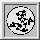Up: Qhull manual: contents
Up: ProgramsOptionsOutputFormatsGeomviewPrintQhullPrecisionTraceFunctions (local)
Up: Qhull code
To: GeomGlobalIoMemMergePolyQhullSetStatUser

## geom_r.c, geom2_r.c, random_r.c -- geometric and floating point routines

Geometrically, a vertex is a point with d coordinates and a facet is a halfspace. A halfspace is defined by an oriented hyperplane through the facet's vertices. A hyperplane is defined by d normalized coefficients and an offset. A point is above a facet if its distance to the facet is positive.

Qhull uses floating point coordinates for input points, vertices, halfspace equations, centrums, and an interior point.

Qhull may be configured for single precision or double precision floating point arithmetic (see realT ).

Each floating point operation may incur round-off error (see Merge). The maximum error for distance computations is determined at initialization. The roundoff error in halfspace computation is accounted for by computing the distance from vertices to the halfspace.

» Geom GlobalIoMemMergePolyQhullSetStatUser

### »geometric data types and constants

• coordT coordinates and coefficients are stored as realT
• pointT a point is an array of DIM3 coordinates

### »mathematical macros

• fabs_ returns the absolute value of a
• fmax_ returns the maximum value of a and b
• fmin_ returns the minimum value of a and b
• maximize_ maximize a value
• minimize_ minimize a value
• det2_ compute a 2-d determinate
• det3_ compute a 3-d determinate
• dX, dY, dZ compute the difference between two coordinates

### »geometric roundoff functions

• qh_detjoggle determine default joggle for points and distance roundoff error
• qh_detmaxoutside determine qh.MAXoutside target for qh_RATIO... tests
• qh_detroundoff determine maximum roundoff error and other precision constants
• qh_distround compute maximum roundoff error due to a distance computation to a normalized hyperplane
• qh_divzero divide by a number that is nearly zero
• qh_maxouter return maximum outer plane
• qh_outerinner return actual outer and inner planesThe Geometry Center Home Page